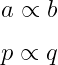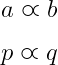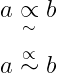# How to write a proportional to symbol( ∝) in LaTeX?

In this tutorial, we will cover proportional to symbol which is a logical operator. In latex, you can define this logical operator using the default and amssymb packages.

Notice the latex program below where the \propto command is used to represent the proportional to symbol.

\documentclass{article}
\begin{document}
$$a \propto b$$
$$p \propto q$$
\end{document}

Output :And you can define proportional to symbols differently using the amssymb package. But, in this case you need to use \varpropto command instead of \propto command.

\documentclass{article}
\usepackage{amssymb}
\begin{document}
$$a \varpropto b$$
$$p \varpropto q$$
\end{document}

Output :In my opinion, using proportional to symbols by default is the best practice.

Many times you will see that proportional to symbol is used with approximate symbol. In this case proportional to and approximate symbols are used together

\documentclass{article}
\usepackage{amsmath}
\begin{document}
$$a \underset{\sim}{\propto} b$$
$$a \stackrel{\propto}{\sim} b$$
\end{document}

Output :#### Md Jidan Mondal

LaTeX expert with over 10 years of experience in document preparation and typesetting. Specializes in creating professional documents, reports, and presentations using LaTeX.

### 2 thoughts on “How to write a proportional to symbol( ∝) in LaTeX?”

1.1.